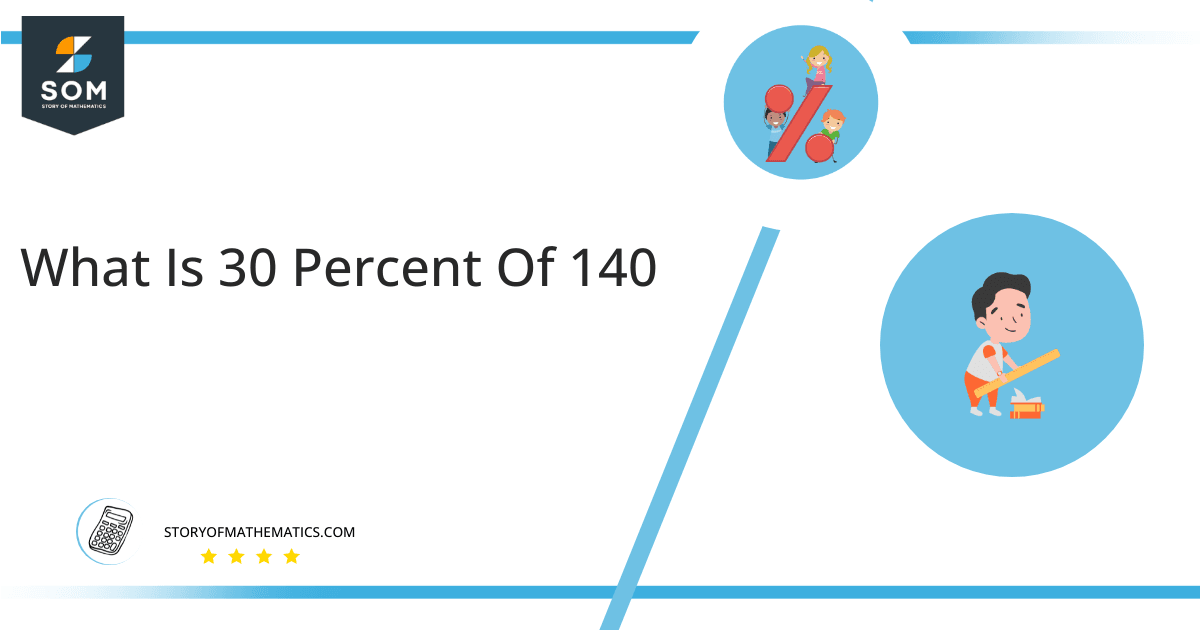# What Is 30 Percent of 140 + Solution with Free Steps?

The 30 percent of 140 is equal to 42. It can be easily calculated by dividing 30 by 100 and multiplying the answer with 140 to get 42.The easiest way to get this answer is by solving a simple mathematical problem of percentages. You need to find 30% of 140 for some sale or real-life problem. Divide 30 by 100, multiply the answer by 140, and get the 30% of 140 value in seconds.

This article will explain the full process of finding any percentage value from any given quantity or number with easy and simple steps.

## What Is 30 percent of 140?

The 30 percent of 140 is 42.

The percentage can be understood with a simple explanation. Take 140, and divide it into 100 equal parts. The 30 parts from the total of 100 parts is called 30 percent, which is 42 in this example.

## How To Calculate 30 percent of 140?

You can find 30 percent of 140 by some simple mathematical steps explained below.### Step 1

Firstly, depict 30 percent of 140 as a fractional multiple as shown below:

30% x 140

### Step 2

The percentage sign % means percent, equivalent to the fraction of 1/100.

Substituting this value in the above formula:

= (30/100) x 140

### Step 3

Using the algebraic simplification process, we can arithmetically manipulate the above equation as follows:

= (30 x 140) / 100

= 4200 / 100

= 42This percentage can be represented on a pie chart for visualization. Let us suppose that the whole pie chart represents the 140 values. Now, we find 30 percent of 140, which is 42. The area occupied by the 42 value will represent the 30 percent of the total 140 values. The remaining region of the pie chart will represent 70 percent of the total 140 values. The 100% of 140 will cover the whole pie chart as 140 is the total value.

Any given number or quantity can be represented in percentages to understand the total quantity better. The percentage can be considered a quantity that divides any number into hundred equal parts for better representation of large numbers and understanding.

Percentage scaling or normalization is a very simple and convenient method of representing numbers in relative terms. Such notations find wide application in many industrial sectors where the relative proportions are used.